## Topic 16.1: Simplex Method (Examples)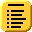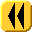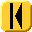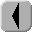Introduction Notes Theory HOWTO Examples Engineering Error Questions Matlab Maple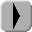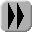# Example 1

Maximize f(x) = 22x1 + 25x2 subject to the constraints:

2x1 + x2 ≤ 26
x1 + x2 ≤ 14
x1 + 2x2 ≤ 22
x1 ≥ 0
x2 ≥ 0

We define the matrix

Chose the first column and perform Gaussian elimination and we note of (26/2 = 13, 14/1 = 14 22/1 = 22) that the 1st entry is the smallest positive value, so we perform Gaussian elimination on the 1st column using the 1st row as a pivot to get

Next, we choose the second column and note that of (13/(1/2) = 26, 1/(1/2) = 2, 9/(3/2) = 6), the second is the smallest, and therefore we continue to use Gaussian elimination on the 2nd column using the 2nd row as a pivot.

The third column still has a negative number, and therefore we check that of (12/1 = 12, 2/(−1) = −2, 6/1 = 6), the third has the smallest positive value, and therefore we perform Gaussian elimination based on that variable:

As both variables were visited, we read off from row one that x1 = 6 and from row two x2 = 8. The value of the objective function is 332.

# Example 2

Use the same system of constraints as in Example 1, but use the objective function f(x) = x1 + 25x2.

In this case, only the 2nd column is in row-reduced echelon form, and therefore x2 = 11 and x1 = 0. The value of the objective function is 275 (= 11 × 25).

# Example 3

Use the same system of constraints as in Example 1, but use the objective function f(x) = 22x1 + x2.

A this point, all indicators are positive, and therefore only one column is reduced, namely, that one corresponding to x1, and therefore our solution is x1 = 13 and x2 = 0, or x = (13, 0)T.